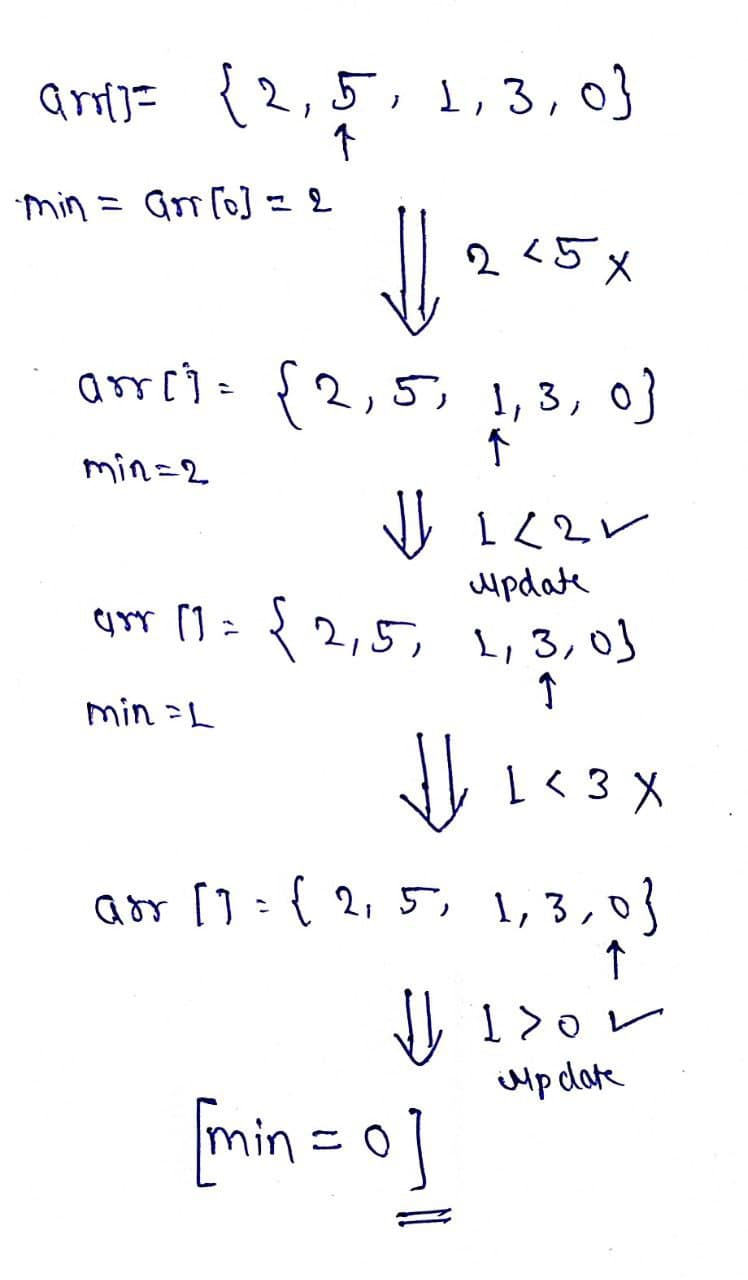# Find the smallest element in an array

Problem Statement: Given an array, we have to find the smallest element in the array.

Examples:

```Example 1:
Input: arr[] = {2,5,1,3,0};
Output: 0
Explanation: 0 is the smallest element in the array.

Example2:
Input: arr[] = {8,10,5,7,9};
Output: 5
Explanation: 5 is the smallest element in the array.```

### Solution

Disclaimer: Don’t jump directly to the solution, try it out yourself first.

Solution1: Sorting

Intuition: We can sort the array in ascending order, hence the smallest element will be at the 0th index.

Approach:

• Sort the array in ascending order.
• Print the 0th index.

DryRun:

Before sorting: arr[] = {2,5,1,3,0};

After sorting: arr[] = {0,1,2,3,5};

Hence answer : arr = 0

Code:

## C++ Code

``````#include<bits/stdc++.h>
using namespace std;

int sortArr(vector<int>& arr) {
sort(arr.begin(),arr.end());
return arr;
}

int main() {
vector<int> arr1 = {2,5,1,3,0};
vector<int> arr2 = {8,10,5,7,9};

cout<<"The smallest element in the array is: "<<sortArr(arr1)<<endl;
cout<<"The smallest element in the array is: "<<sortArr(arr2);

return 0;
}
``````

Output:

The smallest element in the array is: 0
The smallest element in the array is: 5

Time Complexity: O(N*log(N))

Space Complexity: O(1)

## Java Code

``````import java.util.*;
public class tuf {

public static void main(String args[]) {

int arr1[] =  {2,5,1,3,0};
System.out.println("The smallest element in array is: " + sort(arr1));

int arr2[] =  {8,10,5,7,9};
System.out.println("The smallest element in array is: " + sort(arr2));
}
static int sort(int arr[]) {
Arrays.sort(arr);
return arr;
}
}
``````

Output:

The smallest element in the array is: 0
The smallest element in the array is: 5

Time Complexity: O(N*log(N))

Space Complexity: O(1)

Solution2: Using a min variable

Intuition: We can maintain a min variable which will update whenever the current value is less than the value in the min variable.

Approach:

• Create a min variable and initialize it with arr.
• Use a for loop and compare it with other elements of the array
• If any element is less than the min value, update min value with element’s value
• Print the min variable.Code:

## C++ Code

``````#include <iostream>
#include <bits/stdc++.h>

using namespace std;
int SmallestElement(int arr[], int n) {

int min = arr;
for (int i = 0; i < n; i++) {
if (min > arr[i]) {
min = arr[i];
}
}
return min;
}
int main() {
int arr1[] = {2,5,1,3,0};
int n = 5;
int min = SmallestElement(arr1, n);
cout << "The smallest element in the array is: " << min << endl;

int arr2[] =  {8,10,5,7,9};
n = 5;
min = SmallestElement(arr2, n);
cout << "The smallest element in the array is: " << min;
return 0;
}

``````

Output:

The smallest element in the array is: 0
The smallest element in the array is: 5

Time Complexity: O(N)

Space Complexity: O(1)

## Java Code

``````import java.util.*;

public class tuf {

public static void main(String args[]) {

int arr1[] =  {2,5,1,3,0};
System.out.println("The smallest element in the array is: "+SmallestElement(arr1));

int arr2[] =  {8,10,5,7,9};
System.out.println("The smallest element in the array is: "+SmallestElement(arr2));
}
static int SmallestElement(int arr[]) {
int min = arr;
for (int i = 0; i < arr.length; i++) {
if (arr[i] < min) {
min = arr[i];
}
}
return min;
}
}
``````

Output:

The smallest element in the array is: 0
The smallest element in the array is: 5

Time Complexity: O(N)

Space Complexity: O(1)

Special thanks to Prashant Sahu for contributing to this article on takeUforward. If you also wish to share your knowledge with the takeUforward fam, please check out this article